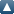# Items where Author is "Al-Mohy, Awad H."Up a level
 Export as ASCII CitationBibTeXDublin CoreEP3 XMLEndNoteHTML CitationJSONMETSMultiline CSVObject IDsOpenURL ContextObjectRDF+N-TriplesRDF+N3RDF+XMLReferReference Manager
Group by: Item Type | No Grouping
Number of items: 27.

## Article

Al-Mohy, Awad H. and Higham, Nicholas J. and Relton, Samuel D. (2015) New Algorithms for Computing the Matrix Sine and Cosine Separately or Simultaneously. SIAM J. Sci. Comput., 37 (1). A456-A487. ISSN 1095-7197

Al-Mohy, Awad H. and Higham, Nicholas J. and Relton, Samuel D. (2013) Computing the Frechet Derivative of the Matrix Logarithm and Estimating the Condition Number. SIAM J. Sci. Comput., 35 (4). C394 -C410. ISSN 1095-7197

Al-Mohy, Awad H. and Higham, Nicholas J. (2012) Improved Inverse Scaling and Squaring Algorithms for the Matrix Logarithm. SIAM Journal on Scientific Computing, 34 (4). C152-C169. ISSN 1095-7197

Al-Mohy, Awad H. and Higham, Nicholas J. (2011) Computing the Action of the Matrix Exponential, with an Application to Exponential Integrators. SIAM Journal on Scientific Computing, 33 (2). pp. 488-511. ISSN 1064-8275

Al-Mohy, Awad H. and Higham, Nicholas J. (2010) The Complex Step Approximation to the Fréchet Derivative of a Matrix Function. Numerical Algorithms, 53 (1). pp. 133-148. ISSN 1017-1398

Higham, Nicholas J. and Al-Mohy, Awad H. (2010) Computing Matrix Functions. Acta Numerica, 19. 159 -208. ISSN 0962-4929

Higham, Nicholas J. and Al-Mohy, Awad H. (2010) Computing Matrix Functions. Acta Numerica, 19. 159 -208. ISSN 0962-4929

Al-Mohy, Awad H. and Higham, Nicholas J. (2009) A New Scaling and Squaring Algorithm for the Matrix Exponential. SIAM Journal On Matrix Analysis and Applications., 31 (3). pp. 970-989. ISSN 1095-7162

Al-Mohy, Awad H. and Higham, Nicholas J. (2009) A New Scaling and Squaring Algorithm for the Matrix Exponential. SIAM Journal On Matrix Analysis and Applications., 31 (3). pp. 970-989. ISSN 1095-7162

Al-Mohy, Awad H. and Higham, Nicholas J. (2009) Computing the Fréchet Derivative of the Matrix Exponential, with an application to Condition Number Estimation. SIAM Journal On Matrix Analysis and Applications., 30 (4). pp. 1639-1657. ISSN 1095-7162

## MIMS Preprint

Al-Mohy, Awad H. (2017) A New Algorithm for Computing the Actions of Trigonometric and Hyperbolic Matrix Functions. [MIMS Preprint]

Al-Mohy, Awad H. (2015) An Efficient Bound for the Condition Number of the Matrix Exponential. [MIMS Preprint]

Al-Mohy, Awad H. and Higham, Nicholas J. and Relton, Samuel D. (2014) New Algorithms for Computing the Matrix Sine and Cosine Separately or Simultaneously. [MIMS Preprint]

Al-Mohy, Awad H. and Higham, Nicholas J. and Relton, Samuel D. (2014) New Algorithms for Computing the Matrix Sine and Cosine Separately or Simultaneously. [MIMS Preprint]

Al-Mohy, Awad H. and Higham, Nicholas J. and Relton, Samuel D. (2012) Computing the Frechet Derivative of the Matrix Logarithm and Estimating the Condition Number. [MIMS Preprint]

Al-Mohy, Awad H. and Higham, Nicholas J. and Relton, Samuel D. (2012) Computing the Frechet Derivative of the Matrix Logarithm and Estimating the Condition Number. [MIMS Preprint]

Al-Mohy, Awad H. and Higham, Nicholas J. and Relton, Samuel D. (2012) Computing the Frechet Derivative of the Matrix Logarithm and Estimating the Condition Number. [MIMS Preprint]

Al-Mohy, Awad H. and Higham, Nicholas J. (2011) Improved Inverse Scaling and Squaring Algorithms for the Matrix Logarithm. [MIMS Preprint]

Al-Mohy, Awad H. and Higham, Nicholas J. (2011) Improved Inverse Scaling and Squaring Algorithms for the Matrix Logarithm. [MIMS Preprint]

Al-Mohy, Awad H. (2011) A More Accurate Briggs Method for the Logarithm. [MIMS Preprint]

Al-Mohy, Awad H. and Higham, Nicholas J. (2010) Computing the Action of the Matrix Exponential, with an Application to Exponential Integrators. [MIMS Preprint]

Al-Mohy, Awad H. and Higham, Nicholas J. (2010) Computing the Action of the Matrix Exponential, with an Application to Exponential Integrators. [MIMS Preprint]

Al-Mohy, Awad H. and Higham, Nicholas J. (2009) The Complex Step Approximation to the Fréchet Derivative of a Matrix Function. [MIMS Preprint]

Al-Mohy, Awad H. and Higham, Nicholas J. (2009) The Complex Step Approximation to the Fréchet Derivative of a Matrix Function. [MIMS Preprint]

Al-Mohy, Awad H. and Higham, Nicholas J. (2009) A New Scaling and Squaring Algorithm for the Matrix Exponential. [MIMS Preprint]

Al-Mohy, Awad H. and Higham, Nicholas J. (2009) A New Scaling and Squaring Algorithm for the Matrix Exponential. [MIMS Preprint]

## Thesis

Al-Mohy, Awad H. (2010) Algorithms for the Matrix Exponential and its Fr\'echet Derivative. Doctoral thesis, University of Manchester.

This list was generated on Mon Sep 26 01:00:52 2022 BST.